[Next]: Research Group Stochastic Algorithms and Nonparametric
 [Up]: Project descriptions
 [Previous]: Stochastic models and numerical algorithms for
[Contents]   [Index]

## Efficient control of stochastic particle methods for rarefied gas flows

Collaborator: W. Wagner , I. Matheis

Cooperation with: S. Ermakov (St. Petersburg University, Russia), A. Garcia (San Jose State University, San Jose, USA), C. Lécot (Université de Savoie, Chambéry, France), O. Muscato (Università di Catania, Italy), S. Rjasanow (Universität des Saarlandes, Saarbrücken)

Supported by: DFG: Effektive Steuerung von stochastischen Partikelverfahren für Strömungen in verdünnten Gasen'' (Effective control of stochastic particle methods for rarefied gas flow)

Description:

Rarefied gas flows play an important role in applications like aerospace design (space shuttle reentry), vacuum engineering (material processing, pumps), or, more recently, nanotechnology. Mathematically, such flows are described (in the simplest case of a monatomic gas) by the Boltzmann equation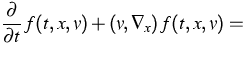(1)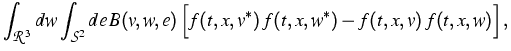where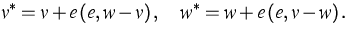(2)
The solution f(t,x,v) represents the relative amount of gas molecules with velocity v at position x and time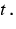The quadratic nonlinearity in (1) corresponds to the pairwise interaction between gas particles, which consists in the change of velocities of two particles according to (2). Here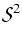denotes the unit sphere in the Euclidean space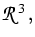and B is called the collision kernel, containing information about the assumed microscopic interaction potential.

Due to the high dimensionality of the problem (f is a function of 7 independent variables) stochastic algorithms are a commonly used tool for the numerical treatment of equation (1). Stochastic particle methods, like the standard DSMC (direct simulation Monte Carlo) technique, are based on the simulation of an appropriate large system of particles approximating the behavior of the real gas. Such methods provide results that are subject to random fluctuations. Thus, the construction of algorithms with reduced fluctuations is an important issue (variance reduction problem). The main purpose of the project is to contribute to this field of research.

In recent years a new approach to the variance reduction problem, called SWPM (stochastic weighted particle method), has been developed (). It uses a system of weighted particles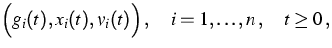where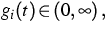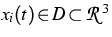and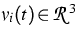denote the weight, position and velocity of the i-th particle at timeThe number of simulation particles is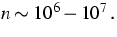The new method has been successfully applied to situations with strong density gradients. In  convergence of the stochastic weighted particle method for the Boltzmann equation was studied. First, the method was extended by introducing new stochastic reduction procedures, in order to control the number of simulation particles. Then, under rather general conditions, convergence to the solution of the Boltzmann equation was proved. Finally, numerical experiments were performed illustrating both convergence and considerable variance reduction, for the specific problem of calculating tails of the velocity distribution.

The application of equation (1) is restricted to flows, where the mean free path between collisions of molecules is sufficiently large compared to the characteristic length scale of the problem. Some modification of the DSMC method, called Consistent Boltzmann Algorithm, has been developed in the literature, extending the field of application into the direction of moderately dense gases. In  the convergence behavior of this algorithm has been studied. The equation was found which is asymptotically solved by the particle system. This equation generalizes the classical Boltzmann equation (1). A further investigation of its relationship to the Enskog equation was the subject of . First an H-theorem for the new equation was established. Then, following the classical derivation by Chapman and Cowling, approximations to the equations of continuity, momentum and energy were found. The first-order correction terms with respect to the particle diameter turned out to be the same as for the Enskog equation.

A kinetic equation, related to quantum gases, was considered in . In this paper a DSMC algorithm for the Uehling-Uhlenbeck-Boltzmann equation is described in terms of Markov processes. This provides a unifying framework for both the classical Boltzmann case as well as the Fermi-Dirac and Bose-Einstein cases. The foundation of the algorithm is established by demonstrating its link to the kinetic equation. By numerical experiments its sensitivity to the number of simulation particles and to the discretization of the velocity space is studied, when approximating the steady state distribution.

References:

1. S. RJASANOW, W. WAGNER, Simulation of rare events by the stochastic weighted particle method for the Boltzmann equation, Math. Comput. Modelling, 33 (2001), pp. 907-926.
2. I. MATHEIS, W. WAGNER, Convergence of the stochastic weighted particle method for the Boltzmann equation, WIAS Preprint no. 739, 2002, to appear in: SIAM J. Sci. Comput.
3. A.L. GARCIA, W. WAGNER, The limiting kinetic equation of the consistent Boltzmann algorithm for dense gases, J. Statist. Phys., 101 (2000), pp. 1065-1086.
4.,Some new properties of the kinetic equation for the Consistent Boltzmann Algorithm, Transport Theory Statist. Phys., 31 (2002), pp. 579-594.
5.,A direct simulation Monte Carlo method for the Uehling-Uhlenbeck-Boltzmann equation, WIAS Preprint no. 763, 2002.

 [Next]: Research Group Stochastic Algorithms and Nonparametric
 [Up]: Project descriptions
 [Previous]: Stochastic models and numerical algorithms for
[Contents]   [Index]

LaTeX typesetting by I. Bremer
5/16/2003